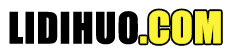# D3.js 数据连接

## 数据连接如何工作？

```[10, 20, 30, 25, 15]
```

### HTML

```<ul id = "list">
<li><li>
<li></li>
</ul>
```

### D3.js 代码

```d3.select("#list").selectAll("li").data([10, 20, 30, 25, 15]);
```

```1. li-10
2. li-20
```

```d3.select("#list").selectAll("li")
.data([10, 20, 30, 25, 15])
.text(function(d) { return d; });
```
text() 方法中的函数用于获取 li 元素的映射数据。这里， d 代表第一个 li 元素为 10，第二个 li 元素为 20。

```3. li-30
4. li-25
5. li-15
```

```d3.select("#list").selectAll("li")
.data([10, 20, 30, 25, 15])
.text(function(d) { return "this is pre-existing element and the value is " + d; })
.enter()
.append("li")
.text(function(d)
{ return "this is dynamically created element and the value is " + d; });
```
Data join 提供了另一种称为 exit() 方法的方法来处理从数据集中动态删除的数据项，如下所示。
```d3.selectAll("li")
.data([10, 20, 30, 15])
.exit()
.remove()
```

```<!DOCTYPE html>
<html>
<script type = "text/javascript" src = "https://d3js.org/d3.v4.min.js"></script>
<style>
body { font-family: Arial; }
</style>
<body>
<ul id = "list">
<li></li>
<li></li>
</ul>

<input type = "button" name = "remove" value = "Remove fourth value"
onclick = "javascript:remove()" />

<script>
d3.select("#list").selectAll("li")
.data([10, 20, 30, 25, 15])
.text(function(d)
{ return "this is pre-existing element and the value is " + d; })
.enter()
.append("li")
.text(function(d)
{ return "this is dynamically created element and the value is " + d; });

function remove() {
d3.selectAll("li")
.data([10, 20, 30, 15])
.exit()
.remove()
}
</script>
</body>
</html>
```

## 数据连接方法

### datum() 方法

datum() 方法用于为 HTML 文档中的单个元素设置值。一旦使用选择器选择元素，它就会被使用。例如，我们可以使用 select() 方法选择现有元素（p 标签），然后使用 datum() 方法设置数据。设置数据后，我们可以更改所选元素的文本或添加新元素并使用 datum() 方法设置的数据分配文本。

```<!DOCTYPE html>
<html>
<script type = "text/javascript" src = "https://d3js.org/d3.v4.min.js"></script>
<body>
<p></p>
<div></div>
<script>
d3.select("p")
.datum(50)
.text(function(d) {
return "Used existing paragraph element and the data " + d + " is assigned.";
});

d3.select("div")
.datum(100)
.append("p")
.text(function(d) {
return "Created new paragraph element and the data " + d + " is assigned.";
});
</script>
</body>
</html>
```

### data() 方法

data() 方法用于将数据集分配给 HTML 文档中的元素集合。一旦使用选择器选择了 HTML 元素，就会使用它。在我们的列表示例中，我们使用它来设置 li 选择器的数据集。
```d3.select("#list").selectAll("li")
.data([10, 20, 30, 25, 15]);
```

### enter() 方法

enter() 方法输出之前不存在图形元素的数据项集。在我们的列表示例中，我们使用它来创建新的 li 元素。
```d3.select("#list").selectAll("li")
.data([10, 20, 30, 25, 15])
.text(function(d) { return "this is pre-existing element and the value is " + d; })
.enter()
.append("li")
.text(function(d) { return "this is dynamically created element and the value is " + d; });
```

### exit() 方法

exit() 方法输出不再存在数据的图形元素集。在我们的列表示例中，我们使用它通过删除数据集中的数据项来动态删除 li 元素之一。
```function remove() {
d3.selectAll("li")
.data([10, 20, 30, 15])
.exit()
.remove()
}
```

## 数据函数

```.text(function(d, i) {
return d;
});
```

```.text(function (d, i) {
console.log(d); // the data element
console.log(i); // the index element
console.log(this); // the current DOM object
return d;
});
```

```<!DOCTYPE html>
<html>
<script type = "text/javascript" src = "https://d3js.org/d3.v4.min.js"></script>
<style>
body { font-family: Arial; }
</style>
<body>
<p></p>
<p></p>
<p></p>
<script>
var data = [1, 2, 3];
var paragraph = d3.select("body")
.selectAll("p")
.data(data)
.text(function (d, i) {
console.log("d: " + d);
console.log("i: " + i);
console.log("this: " + this);
return "The index is " + i + " and the data is " + d;
});
</script>
</body>
</html>
```